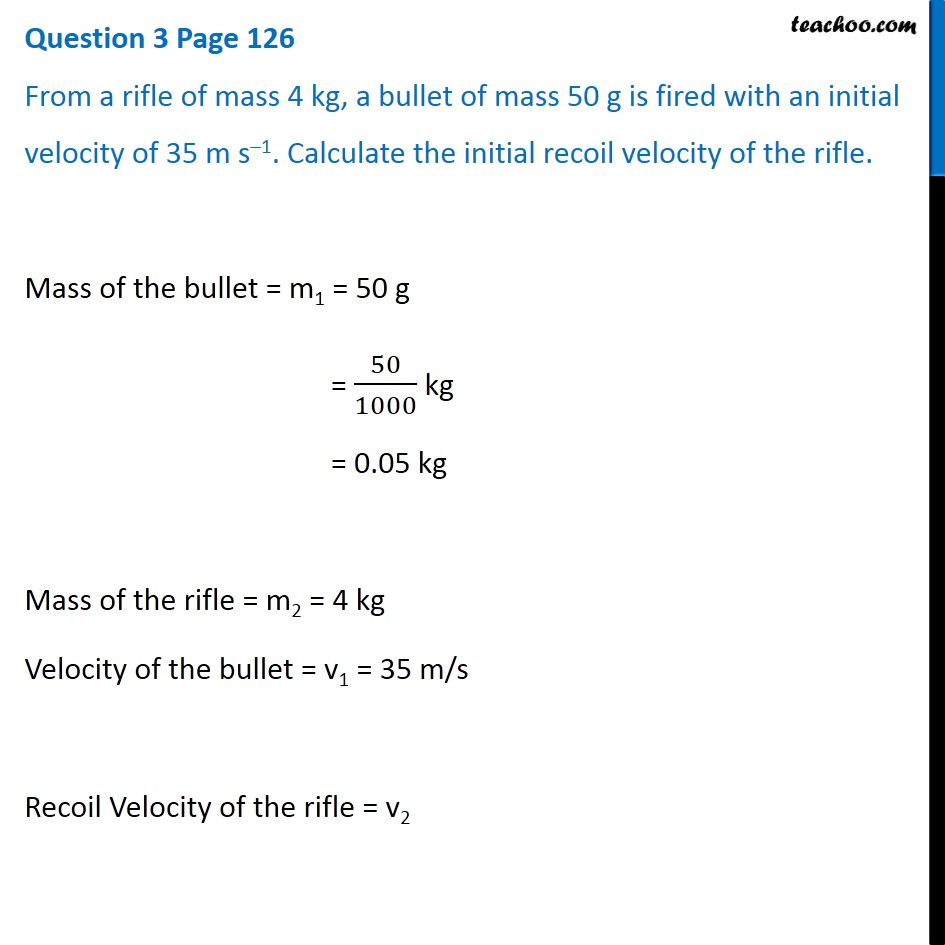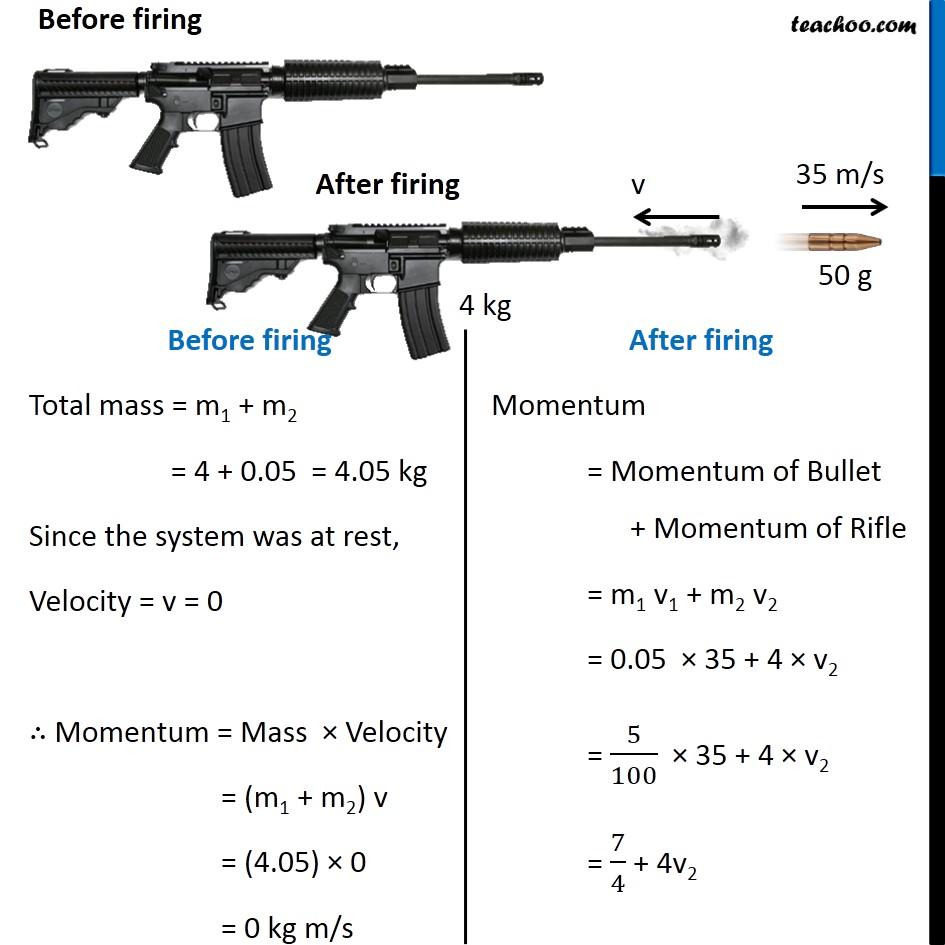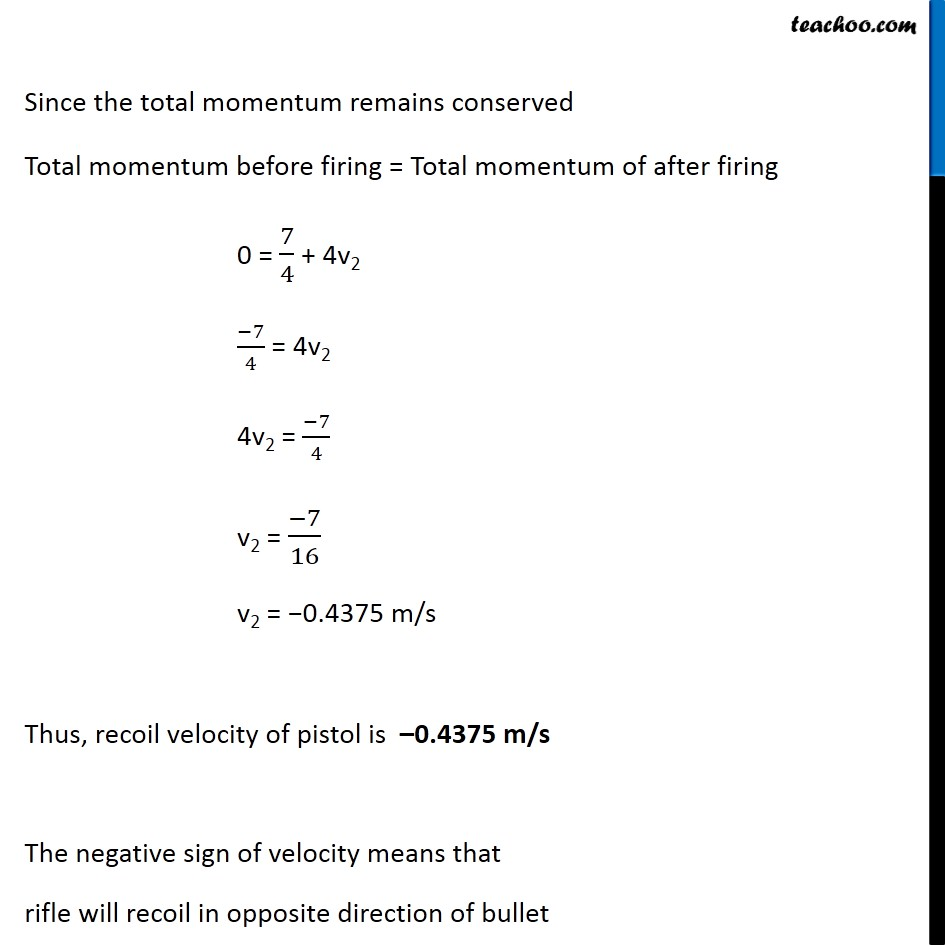Questions from inside the chapter

Class 9
Chapter 9 Class 9 - Force and Laws Of MotionLearn in your speed, with individual attention - Teachoo Maths 1-on-1 Class

### Transcript

Question 3 Page 126 From a rifle of mass 4 kg, a bullet of mass 50 g is fired with an initial velocity of 35 m s–1. Calculate the initial recoil velocity of the rifle. Mass of the bullet = m1 = 50 g = 50/1000 kg = 0.05 kg Mass of the rifle = m2 = 4 kg Velocity of the bullet = v1 = 35 m/s Recoil Velocity of the rifle = v2 Before firing After firing Before firing Total mass = m1 + m2 = 4 + 0.05 = 4.05 kg Since the system was at rest, Velocity = v = 0 ∴ Momentum = Mass × Velocity = (m1 + m2) v = (4.05) × 0 = 0 kg m/s After firing Momentum = Momentum of Bullet + Momentum of Rifle = m1 v1 + m2 v2 = 0.05 × 35 + 4 × v2 = 5/100 × 35 + 4 × v2 = 7/4 + 4v2 After firing Momentum = Momentum of Bullet + Momentum of Rifle = m1 v1 + m2 v2 = 0.05 × 35 + 4 × v2 = 5/100 × 35 + 4 × v2 = 7/4 + 4v2 After firing Momentum = Momentum of Bullet + Momentum of Rifle = m1 v1 + m2 v2 = 0.05 × 35 + 4 × v2 = 5/100 × 35 + 4 × v2 = 7/4 + 4v2 Since the total momentum remains conserved Total momentum before firing = Total momentum of after firing 0 = 7/4 + 4v2 (−7)/4 = 4v2 4v2 = (−7)/4 v2 = (−7)/16 v2 = −0.4375 m/s Thus, recoil velocity of pistol is –0.4375 m/s The negative sign of velocity means that rifle will recoil in opposite direction of bullet